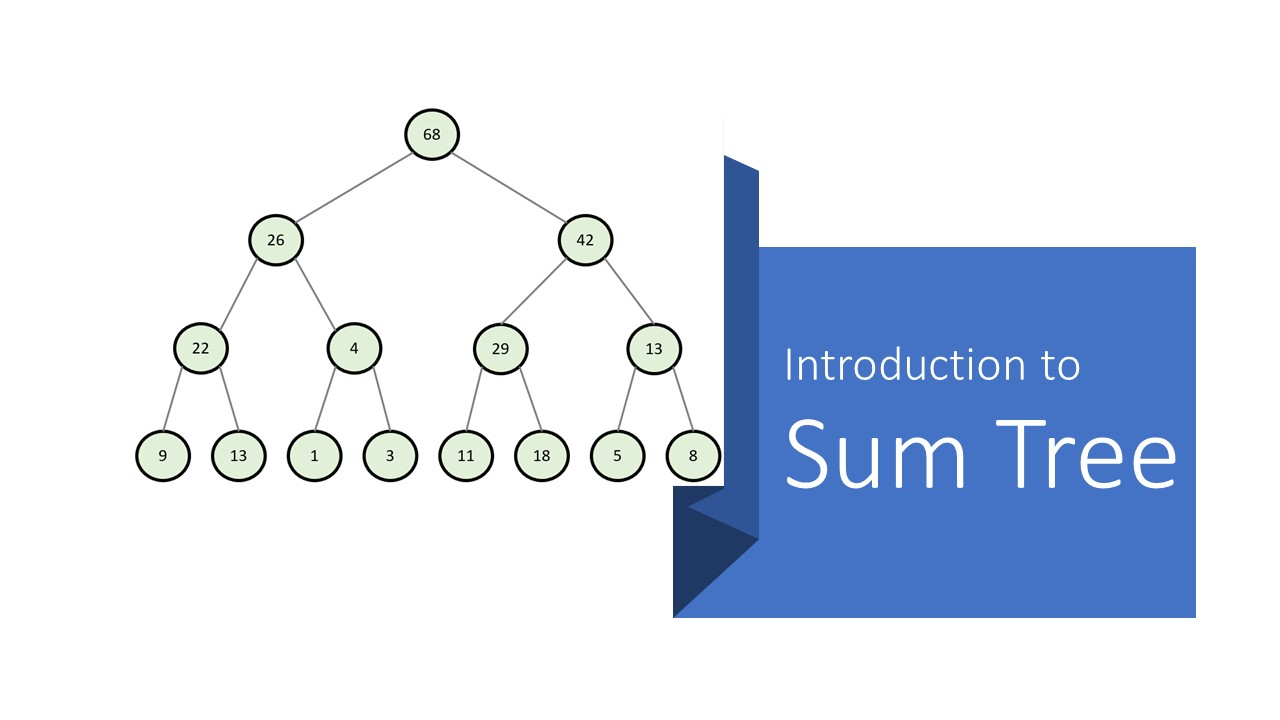Code

### Introduction to Sum Tree

October 31, 2021
5 min
By
RameshShare

#### What is a Sum Tree ?

Sum Tree is a binary tree. A binary tree is a tree data structure where each parent node has a maximum of 2 child nodes. Sum tree is a special kind of binary tree where the value of a parent node is equal to the sum of the values of its children.

#### Why Sum Trees ?

Suppose you have a list of data and you need to sample the data from the list randomly. One thing that you could do is generate a random integer between zero and size of the list and retrieve data from the list.

With this method, you will be able to get data from the list in an equiprobable manner.

What if you need to get some data out of the list with a higher priority. Can you think of a way to retrieve data from a list randomly, which gives a high chance of retrieval for some the high priority data?

You can try to sort the data list with the priority values from high to low. Then what you need is to get a random number from a generator which has a high chance to give a number close to zero and low chance when the number is far away from zero. Probably a Gaussian random number generator with mean equals to zero would do the thing. You can adjust the sigma to accordingly.

The main problem here is how to adjust the sigma to fit the size of the list. If the size of the list is increased, then you will need to adjust sigma again. Also if the generator gives a number which is higher than the size of the list, what to do?
So this method would be not feasible.

You can try this. You can generate a random number between zero and sum of total priority values in the data list (in the example it is 1012.4) from a uniform distribution. Let’s say you got the number 430.58 from the random number generator. Then you have to sum the priority values of the list from left to right until the sum exceeds 430.58.

In the above example, the sum of the first 24 elements is 427.7 and the sum of all the first 25 elements is 442.8. So the 25th element is the element that you need to retrieve from the list.

If this is hard to understand, lets try with a small example.

In this case, you have a total probability of 68. Now you need to generate a random number from a uniform distribution. If the generated number is between 0-17, you will have to select the first element from the list. If it is between 18-30, you have to select the second element, and so on. Now you can understand that there is a higher chance to retrieve the first element from the data list.

If you think about this more, you will understand that you don’t even need to sort the data list. Without sorting if you follow the summing method, you will have a higher chance to retrieve the elements with higher probability values.

This method is interesting, right? But it has a complexity of $\mathcal{O}(n)$ while retrieving data. If you have a large list of data, this method will be really painful. That’s when the Sum Tree comes to the picture. We can store the data in a Sum Tree and retrieve accordingly with only a complexity of $\mathcal{O}(\log{n})$.

In a Sum Tree, you can store only a fixed number of data. You have to decide that in the beginning. Let’s say that you to store 8 data values with priorities in a Sum Tree. Then you will need a tree with 15 (=8+7) nodes where 8 nodes are leaves that store the priority values and other 7 are the parent nodes. If you store the above data list in a Sum Tree, the result would be like the following. You will see that each parent value is equal to the sum of the values of its children.

Data retrieval pseudocode will be as follows.

If you give the root node and a random number between zero and total priority to this function, it will return the leaf node with the corresponding priority. From that leaf node, you can retrieve the corresponding data from the list.

In the above example, lets say you got the number 24 from the random number generator. Then the algorithm will return the following node.

It’s easy…

Maybe a python implementation of Sum Tree will be explained in a future article. Stay Tuned!!